AtomicDensityMatrix`
AtomicDensityMatrix`

# HyperfineB

HyperfineB

is a parameter for AtomicState that parameterizes the hyperfine splitting of the state due to the electronic interaction with the nuclear electric quadrupole moment.

HyperfineB[state]

returns the value specified for HyperfineB in AtomicState state, or HyperfineB[StateLabel[state]] if it is not specified.

# Details and Options

• HyperfineB is considered to have units of angular frequency.
• The hyperfine shift of a state with HyperfineA and HyperfineB denoted by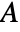and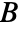, respectively, is given by, where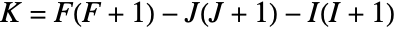and,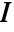, and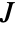are the total, total electronic, and total nuclear angular-momentum quantum numbers, respectively.
• HyperfineB is automatically threaded over a list of AtomicState objects appearing as its argument.

# Examples

## Basic Examples(1)

Specify the HyperfineA and HyperfineB parameters for a state with hyperfine structure, omitting the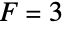sublevel from the system:

Get the specified HyperfineB parameters for each Zeeman sublevel:

The values of HyperfineA and HyperfineB are used to calculate the hyperfine shift for each sublevel: